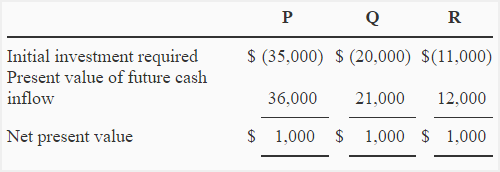# Exercise-5 (Present value index – ranking investment projects)

The management of National company is considering three competing investments – investment P, investment Q and investment R. The information about the requirement of initial amount of investment, present value of net cash inflow and net present value of all three investments is given below:Required:

Choose the most desirable proposal using present value index (profitability index).

## Solution:

As each investment requires a different initial investment, the proposals would be ranked using present value index (also called profitability index).

Present value index = Present value of cash inflows/Initial investment

Proposal P: \$36,000/\$35,000 = 1.03

Proposal Q: \$21,000/\$20,000 = 1.05

Proposal R: \$12,000/\$11,000 = 1.09

Proposal R is the most desirable proposal because it has the highest present value index.

A D V E R T I S E M E N T
4 Comments on Exercise-5 (Present value index – ranking investment projects)
1.jeniffer

Need help in calculating this quiz.
angola company has six projects available for investment as follows.
project initial cost sh’m’ [email protected] 15% cost of cap.
1 60 21
2 15 9
3 20 9
4 55 15
5 30 20
6 40 -2

the firm has sh.100m available for investment. identify which project should be undertaken. using PI and NPV.

1.Njohnny

If we divide the present value of future cash inflow by the initial investment, we would get the Present Value Index, so,
Present value Index would be :
1-: 60/ 21 =2.857
2-: 15/ 9 =1.667
3-: 20/ 9 =2.222
4-: 55/ 15 =3.667
5-: 30/ 20 =1.50
6-: 40/ -2 =-20
The proposal 4 would be the most desirable because of the highest present value index. or profitability index of 3.667. Which maybe higher than what the management foresaw.

Now , the net present value would be : 55-15 =40.
So according to the proposal 4, the Net Present Value is 40.

2.Rohit

PvP factor 20% Korma hoga
31300 initial outlay h or invest amount 100000 h reply fast mera ‘kl paper h
annual cash flow 6000 h

3.Kim

Question
A firm has to choose between these possible projects and the details of each project are as follows:

Capital cost \$
Project A 300,000
Project B 500,000
Project C 450,000

Net cash inflow
Project A Project B Project C
Year 1 75,000 100,000 50,000
Year 2 125,000 200,000 75,000
Year 3 125,000 300,000 250,000
Year 4 100,000 300,000 300,000
Year 5 75,000 150,000 200,000
What is the Payback period – Project A, B, & C
Calculate the AAR for each of the projects
Which project would you recommend to the board to accept and why?